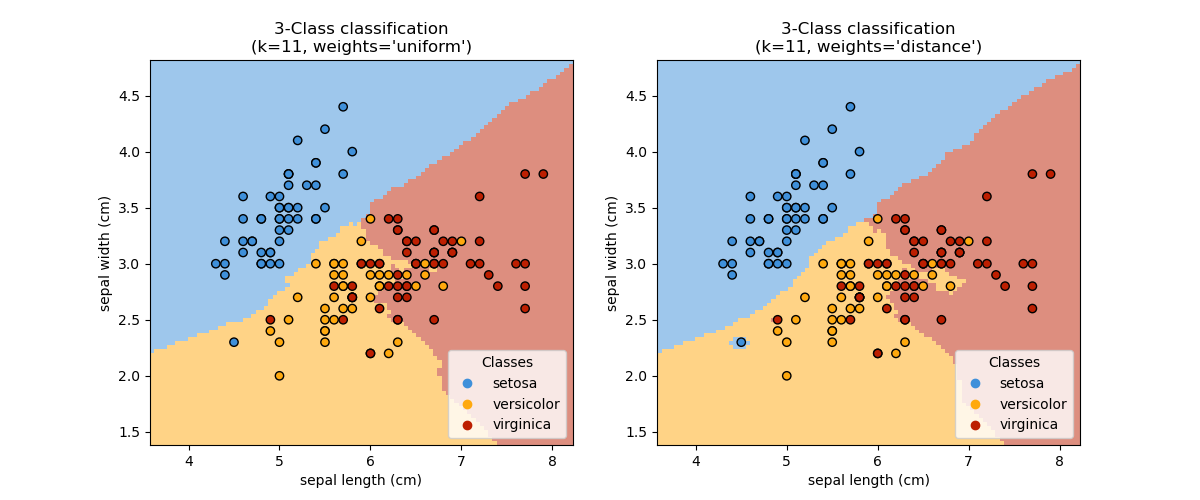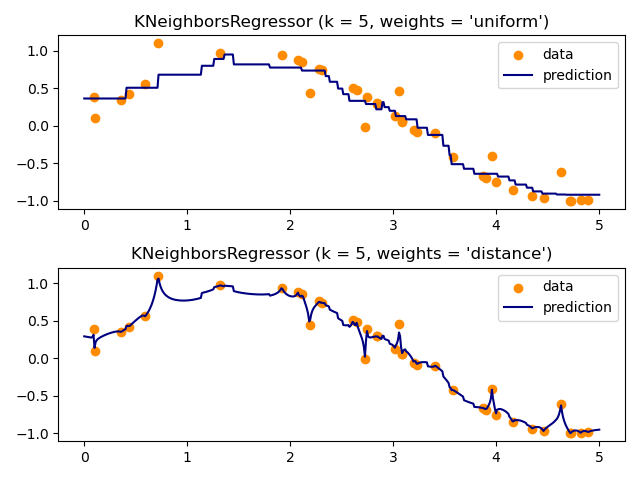Anthony Lee

kNN(k Nearest Neighbors) Algorithm

IntroductionAnthony Lee

I love to code and better the world. Graduate student at Georgia Tech specializing in Machine Learning.

Featured

kNN(k Nearest Neighbors) Algorithm

Posted by Anthony Lee on .

kNN is one of the algorithm used for classification and regression in Supervised Learning. It is regarded as one of the simplest machine learning algorithm.

Unlike other Supervised Learning algorithms, it does not have a training phase. The training and testing is pretty much the same thing. It is a lazy learner where training dataset is already stored. Because of that very reason, kNN is not an ideal candidate for algorithm that needs to process large data set.

With kNN, you are basically looking for the closest points to the new point. The k represents the amount of nearest neighbors of the unknown point. We provide the k amount (Often an odd number) of the algorithm to predict the outcome.

• kNN is a type of instance-based learning, or lazy learning, where the function is only approximated locally and all computation is deferred until classification.
• The kNN algorithm is among the simplest of all Machine Learning Algorithms.
• In kNN classification, the output is a class membership. An Object is classified by a majority vote of its neighbors, with the object being assigned to the class most common among its k nearest neighbors (k is a positive integer, typically small).

Reference: https://en.wikipedia.org/wiki/K-nearest_neighbors_algorithm

What does it measure?
Euclidean Distance.You can write this in Python like this: math.sqrt((x2-x1)**2 + (y2-y1)**2)

Pros and Cons?
Pros: High accuracy, insensitive to outliers, no assumptions about data.
Cons: Computationally expensive, high memory requirement.
Works with: Numeric values, nominal values.

Good tools?
Scikit-learn is a great Machine Learning library to perform machine learning algorithm.

Example of kNN classification example from scikit:Example of kNN regression example from scikit:Anthony Lee

http://anthonylee.io

I love to code and better the world. Graduate student at Georgia Tech specializing in Machine Learning.